# Engineering Mechanics Dynamics 14th Ed. I need problem 24p from chapter 12. I have orderd the...

Engineering Mechanics Dynamics 14th Ed. I need problem 24p from chapter 12. I have orderd the book but it hasnt come in yet. I have the answer but I still need the actual problem from the book. Thanks.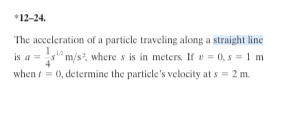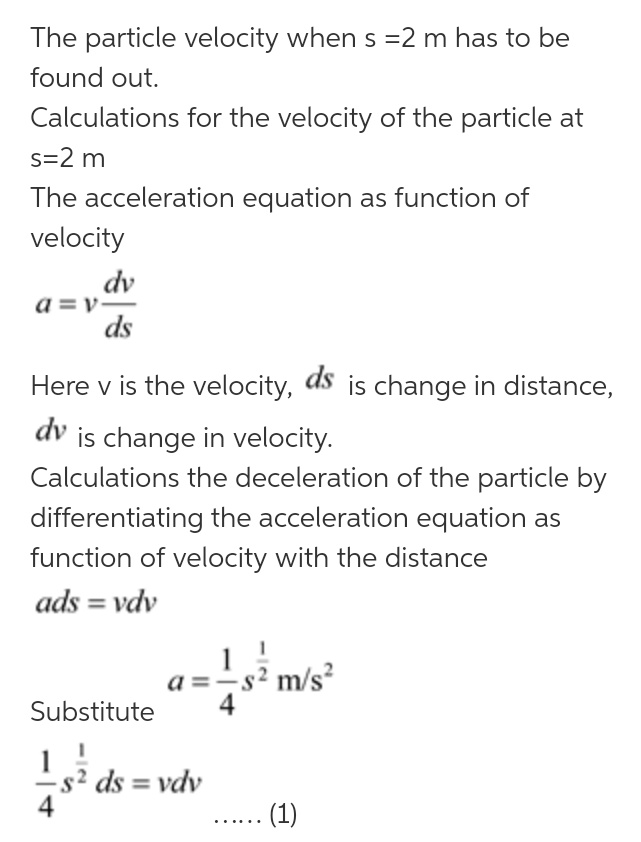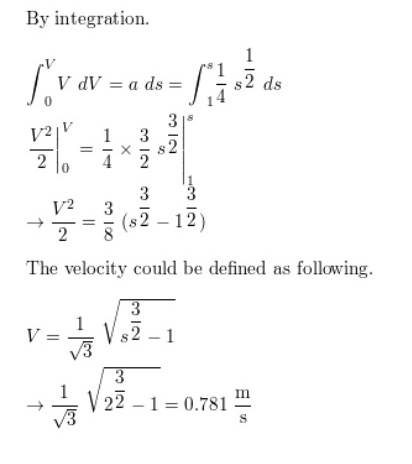#### Earn Coin

Coins can be redeemed for fabulous gifts.

Similar Homework Help Questions
• ### Engineering Mechanics Dynamics Please read all. Especially the highlighted area. I still need a step by...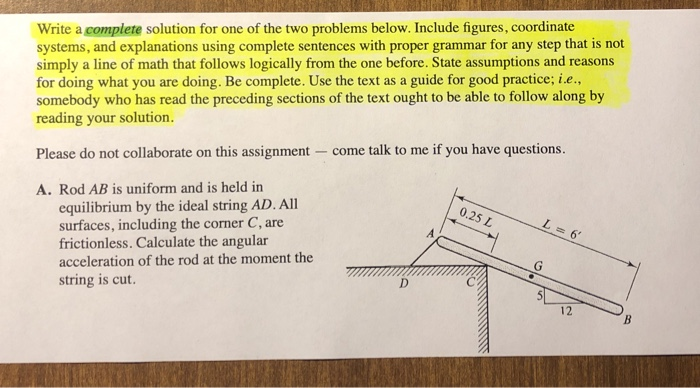Engineering Mechanics Dynamics Please read all. Especially the highlighted area. I still need a step by step calculation. Of the problem. This is a final test question. Write a complete solution for one of the two problems below. Include figures, coordinate systems, and explanations using complete sentences with proper grammar for any step that is not simply a line of math that follows logically from the one before. State assumptions and reasons for doing what you are doing. Be complete....

• ### Meriam, Engineering Mechanics: Dynamics, 9e ANALYTIC MECHANICS- DYNAMICS (ENGR I Chapter 3, P A 75-kg woman...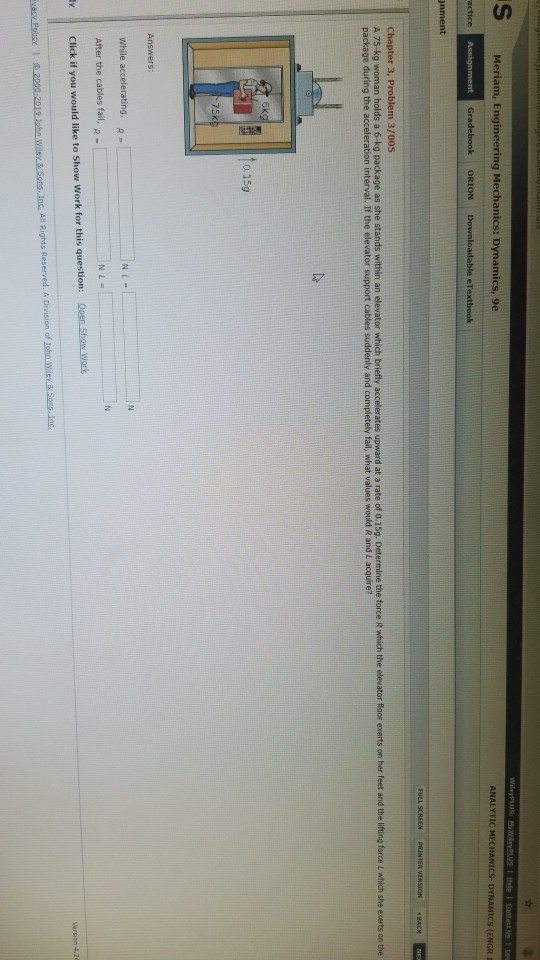Meriam, Engineering Mechanics: Dynamics, 9e ANALYTIC MECHANICS- DYNAMICS (ENGR I Chapter 3, P A 75-kg woman holds a 6-kg package as she stands within an elevator which briefly accelerates upward at a rate of 0.15g. D package during the acceleration interval. If the elevator support cables suddenly and completely fail, what values would R and i acquire? 3/005 0.15g While accelerating, R After the cables fail, R- Click if you would like to Show Work for this question: Oeen Show...

• ### Chapter 6, Problem 147P from Vector Mechanics for Engineers: Statics and Dynamics (7th) by Beer,...

I would like to know if I can have the solution for Problem147P, Chapter 6. The book is Vector Mechanics for Engineers:Statics and Dynamics (7th) by Beer Johnston.Thanks,Duffy

• ### Problem 17.93 from Engineering Mechanics: Statics and Dynamics 12th Edition by R.C. Hibbeler

PLEASE POST SOLUTION for problem 17.93 from Engineering Mechanics: Statics and Dynamics 12th Edition by R.C. Hibbeler

• ### Vector Mechanics for Engineers: Statics and Dynamics (8th) Chapter 5 Problem 35P

Hello, Dear helper, I need the solutionfor Vector Mechanics for Engineers: Statics and Dynamics (8th) Chapter 5 Problem 35P. I thank you always your help. If Iget this question solution as soon as possible I really appreciated. thank you again!

• ### ENGINEERING MECHANICS ?-ESC204-DYNAMICS TEST Freeman Summer 2018 Problem 3 (15 points) (chapter 13 problem) A 400-kg...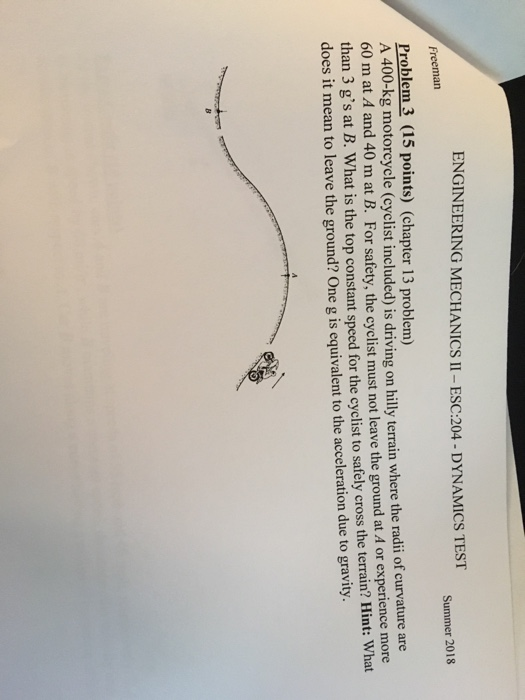ENGINEERING MECHANICS ?-ESC204-DYNAMICS TEST Freeman Summer 2018 Problem 3 (15 points) (chapter 13 problem) A 400-kg motorcycle (cyclist included) is driving on hlly terrain where the radii of curvature are 60 m at A and 40 m at B. For safety, the cyclist must not leave the ground at A or experience more than 3 g's at B. What is the top constant speed for the cyclist to safely cross the terrain? Hint: What does it mean to leave the...

• ### Meriam, Engineering Mechanics: Dynamics, 9e Help | System Announcements PRINTER VERSION BACK NEXT > Chapter 3,...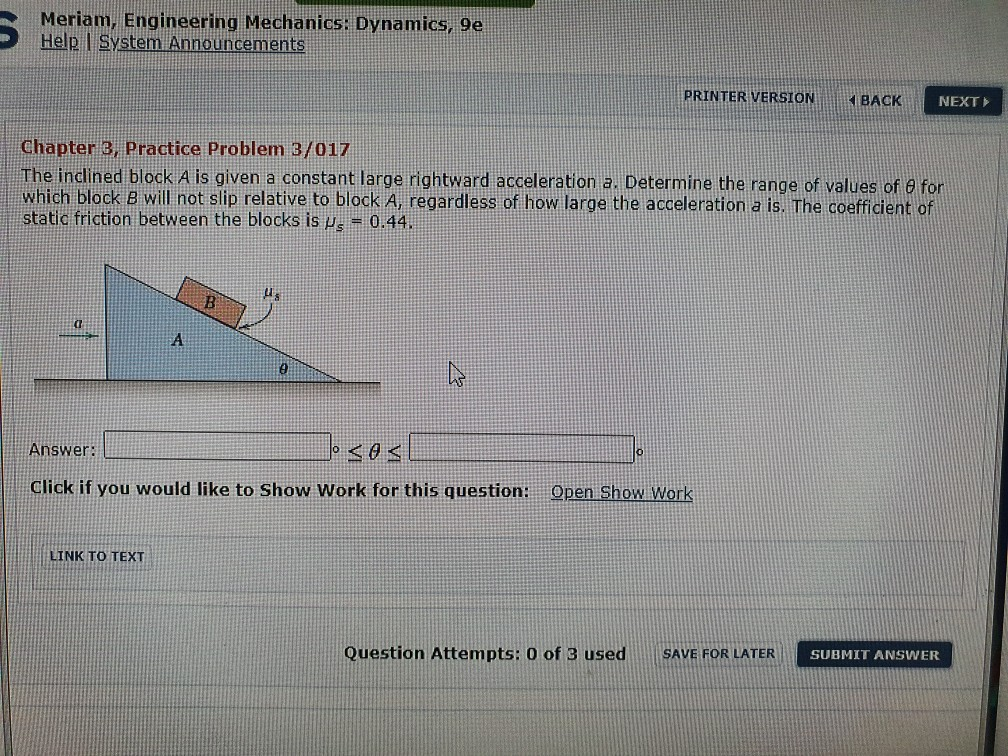Meriam, Engineering Mechanics: Dynamics, 9e Help | System Announcements PRINTER VERSION BACK NEXT > Chapter 3, Practice Problem 3/017 The inclined block A is given a constant large rightward acceleration a. Determine the range of values of e for which block B will not slip relative to block A, regardless of how large the acceleration a is. The coefficient of static friction between the blocks is us = 0.44. B А e Answer: < Ꮎ < Click if you would...

• ### Vector Mechanics for Engineers: Dynamics 9th Edition problem 12-14

Hello,I am looking for the solution to Vector Mechanics for Engineers: Dynamics 9th Edition Chapter 12 problem 14. Here is is:A tractor trailer is traveling at 60 mi/h when the driver applies his brakes. Knowing that the braking fore of the tractor and the trailer are 3600 lb and 13,700,respectively, determine (a) the distance traveled by the tractor trailer before it comes to a stop, (b) the horizontal component of the force in the hitch between thetractor and the trailer...

• ### Problem 3.81 "Vector Mechanics for Engineers"-Statics

Cramster,The following question is from the book: "Vector Mechanics forEngineers"....Statics. The problem number is in Chapter 3,#81.Problem Statement: A tubboat exerts a 2.8-kN force along itsaxis on a barge at point B. Replace the force applied at B with anequivalent system formed by twoparallel forces applied at A andC.BARGEABCboatThe tug boat applies its force at a 65 deg angle from thepositive x-axis at point B. The distance between A and B is 27 m,and the distance between B and C...

• ### I need a solution for this problem with extra details The book is Goldstein classical mechanics...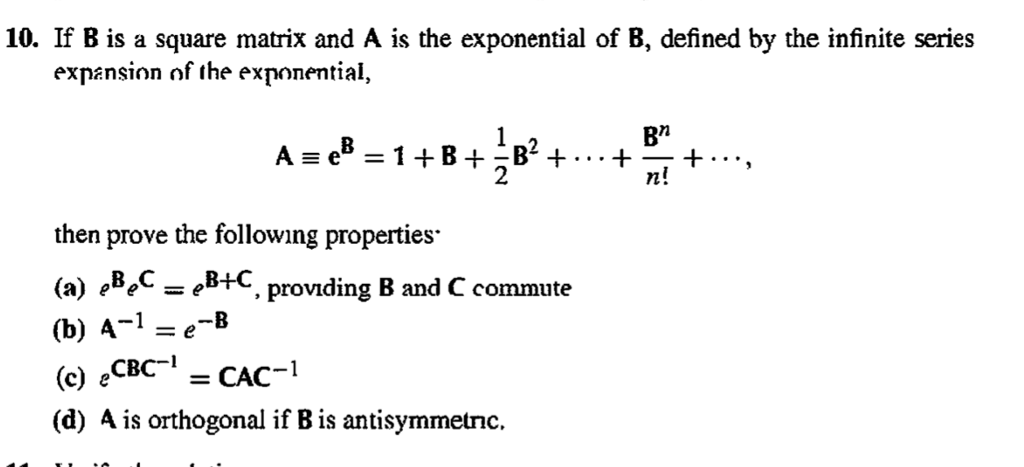I need a solution for this problem with extra details The book is Goldstein classical mechanics 3rd edition and the chapter   4.10 10. If B is a square matrix and A is the exponential of B, defined by the infinite series expansion of the exnonential, As e 1+B+B2+. . +-+..., ?" n! 2 then prove the following properties (a) eLeC-eB+C, prov?ding B and C commute (b) A-1-e-B (c) e (d) A is orthogonal if B is antisymmetnc. CBC1 CAC